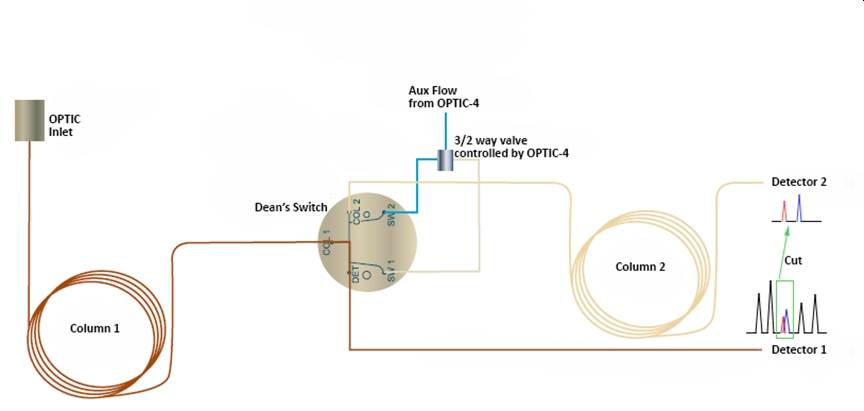$$\newcommand{\vecs}{\overset { \rightharpoonup} {\mathbf{#1}} }$$ $$\newcommand{\vecd}{\overset{-\!-\!\rightharpoonup}{\vphantom{a}\smash {#1}}}$$$$\newcommand{\id}{\mathrm{id}}$$ $$\newcommand{\Span}{\mathrm{span}}$$ $$\newcommand{\kernel}{\mathrm{null}\,}$$ $$\newcommand{\range}{\mathrm{range}\,}$$ $$\newcommand{\RealPart}{\mathrm{Re}}$$ $$\newcommand{\ImaginaryPart}{\mathrm{Im}}$$ $$\newcommand{\Argument}{\mathrm{Arg}}$$ $$\newcommand{\norm}{\| #1 \|}$$ $$\newcommand{\inner}{\langle #1, #2 \rangle}$$ $$\newcommand{\Span}{\mathrm{span}}$$ $$\newcommand{\id}{\mathrm{id}}$$ $$\newcommand{\Span}{\mathrm{span}}$$ $$\newcommand{\kernel}{\mathrm{null}\,}$$ $$\newcommand{\range}{\mathrm{range}\,}$$ $$\newcommand{\RealPart}{\mathrm{Re}}$$ $$\newcommand{\ImaginaryPart}{\mathrm{Im}}$$ $$\newcommand{\Argument}{\mathrm{Arg}}$$ $$\newcommand{\norm}{\| #1 \|}$$ $$\newcommand{\inner}{\langle #1, #2 \rangle}$$ $$\newcommand{\Span}{\mathrm{span}}$$$$\newcommand{\AA}{\unicode[.8,0]{x212B}}$$

### Multidimensional Gas Chromatography (GC-GC, 2D-GC)

Recently the idea of multidimensional gas chromatography separations has be proven to be very useful for closely eluting solutes  in experiments with a single column. (see Liu and Phillips, Journal of Chromatographic Science. 29 (6), 227–231, 1991).  Multidimensional GC is based upon sending groups of closely eluting solutes (on a long column of one selectivity onto a second shorter column of a different selectivity).  As shown in Figure$$\PageIndex{1}$$ a fast value is used to the  red and blue co-eluting in Column 1 solutes onto Column 2 where are being further separated they are better separated.Figure$$\PageIndex{1}$$ : A schematic diagram of a two dimension GC instrument.  Image source currently unknow.

An example of the utility of multidimensional GC is shown in Figure$$\PageIndex{2}$$ - Figure$$\PageIndex{4}$$.  Figure$$\PageIndex{2}$$ depicts a typical one dimensional GC separation of a large number of polycyclic aromatic compounds on an appropriate coluum.  Despite the 40 m length of the capillary column, there are numerous examples of co-eluting solutes presented in the focus boxes.Figure$$\PageIndex{2}$$ : The gas chromatograph for a large series of polycyclic aromoatic solutes on a 40 M RXi-PAH column.  Imge source currently unknown

Figure$$\PageIndex{3}$$ reveals the 2D chromatogram when the solutes eluting through the RXi-PAH column arriving between approximately 15 and 24 min in Figure$$\PageIndex{1}$$ are directed onto a short, 1 M, RXi-1HT column for separation on a column with a different selectivity.  (Note an even longer RXi-PAH column, 60 M, was used in the the 2D GC instrument)Figure$$\PageIndex{3}$$:  A focused region in the 2D - GC experiment of the solutes eluting from approximately 15 min to 24 min in Figure 12.2.2.  Image source currently unknown.

Figure$$\PageIndex{4}$$ is a zoom of the region in Figure 12.2.3 corresponding to the box centered at 16 min in Figure$$\PageIndex{2}$$ and containing the signals for benz[a]anthracene, chrysene, triphenylene and cyclopenta[cd]pyrene revealing the full resolution for the separation of these solutes.ht

Figure$$\PageIndex{4}$$ : A focused region in the 2D - GC experiment revealing the full resolution of the following solutes: benz[a]anthracene, chrysene, triphenylene and cyclopenta[cd]pyrene.  Image source currently unknown.

13.2: Advances in GC is shared under a not declared license and was authored, remixed, and/or curated by LibreTexts.